·  Page d'accueil  ·  Toutes les classes  ·  Toutes les fonctions  ·  Vues d'ensemble  ·

# QML PropertyAnimation Element

The PropertyAnimation element animates changes in property values. More...

Inherits Animation

Inherited by ColorAnimation, NumberAnimation, RotationAnimation, and Vector3dAnimation.

This element was introduced in Qt 4.7.

## Detailed Description

PropertyAnimation provides a way to animate changes to a property's value.

It can be used to define animations in a number of ways:

• In a Transition

For example, to animate any objects that have changed their x or y properties as a result of a state change, using an InOutQuad easing curve:

``` Rectangle {
id: rect
width: 100; height: 100
color: "red"

states: State {
name: "moved"
PropertyChanges { target: rect; x: 50 }
}

transitions: Transition {
PropertyAnimation { properties: "x,y"; easing.type: Easing.InOutQuad }
}
}```
• In a Behavior

For example, to animate all changes to a rectangle's x property:

``` Rectangle {
width: 100; height: 100
color: "red"

Behavior on x { PropertyAnimation {} }

MouseArea { anchors.fill: parent; onClicked: parent.x = 50 }
}```
• As a property value source

For example, to repeatedly animate the rectangle's x property:

``` Rectangle {
width: 100; height: 100
color: "red"

SequentialAnimation on x {
loops: Animation.Infinite
PropertyAnimation { to: 50 }
PropertyAnimation { to: 0 }
}
}```
• In a signal handler

For example, to fade out theObject when clicked:

``` MouseArea {
anchors.fill: theObject
onClicked: PropertyAnimation { target: theObject; property: "opacity"; to: 0 }
}```
• Standalone

For example, to animate rect's width property over 500ms, from its current width to 30:

``` Rectangle {
id: theRect
width: 100; height: 100
color: "red"

// this is a standalone animation, it's not running by default
PropertyAnimation { id: animation; target: theRect; property: "width"; to: 30; duration: 500 }

MouseArea { anchors.fill: parent; onClicked: animation.running = true }
}```

Depending on how the animation is used, the set of properties normally used will be different. For more information see the individual property documentation, as well as the QML Animation and Transitions introduction.

Note that PropertyAnimation inherits the abstract Animation element. This includes additional properties and methods for controlling the animation.

## Property Documentation

 duration : int

This property holds the duration of the animation, in milliseconds.

The default value is 250.

 easing.type : enumeration easing.amplitude : real easing.overshoot : real easing.period : real

To specify an easing curve you need to specify at least the type. For some curves you can also specify amplitude, period and/or overshoot (more details provided after the table). The default easing curve is Easing.Linear.

` PropertyAnimation { properties: "y"; easing.type: Easing.InOutElastic; easing.amplitude: 2.0; easing.period: 1.5 }`

Available types are:

 Easing.Linear Easing curve for a linear (t) function: velocity is constant.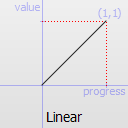Easing.InQuad Easing curve for a quadratic (t^2) function: accelerating from zero velocity.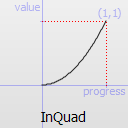Easing.OutQuad Easing curve for a quadratic (t^2) function: decelerating to zero velocity.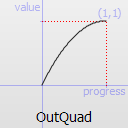Easing.InOutQuad Easing curve for a quadratic (t^2) function: acceleration until halfway, then deceleration.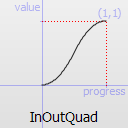Easing.OutInQuad Easing curve for a quadratic (t^2) function: deceleration until halfway, then acceleration.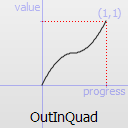Easing.InCubic Easing curve for a cubic (t^3) function: accelerating from zero velocity.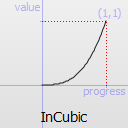Easing.OutCubic Easing curve for a cubic (t^3) function: decelerating from zero velocity.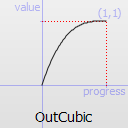Easing.InOutCubic Easing curve for a cubic (t^3) function: acceleration until halfway, then deceleration.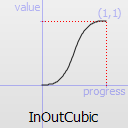Easing.OutInCubic Easing curve for a cubic (t^3) function: deceleration until halfway, then acceleration.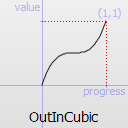Easing.InQuart Easing curve for a quartic (t^4) function: accelerating from zero velocity.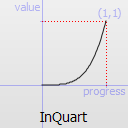Easing.OutQuart Easing curve for a quartic (t^4) function: decelerating from zero velocity.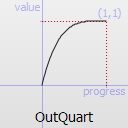Easing.InOutQuart Easing curve for a quartic (t^4) function: acceleration until halfway, then deceleration.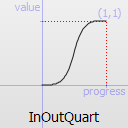Easing.OutInQuart Easing curve for a quartic (t^4) function: deceleration until halfway, then acceleration.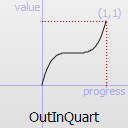Easing.InQuint Easing curve for a quintic (t^5) function: accelerating from zero velocity.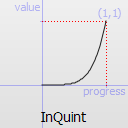Easing.OutQuint Easing curve for a quintic (t^5) function: decelerating from zero velocity.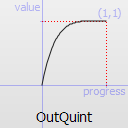Easing.InOutQuint Easing curve for a quintic (t^5) function: acceleration until halfway, then deceleration.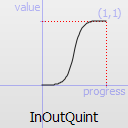Easing.OutInQuint Easing curve for a quintic (t^5) function: deceleration until halfway, then acceleration.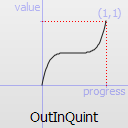Easing.InSine Easing curve for a sinusoidal (sin(t)) function: accelerating from zero velocity.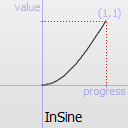Easing.OutSine Easing curve for a sinusoidal (sin(t)) function: decelerating from zero velocity.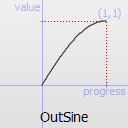Easing.InOutSine Easing curve for a sinusoidal (sin(t)) function: acceleration until halfway, then deceleration.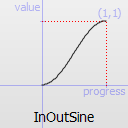Easing.OutInSine Easing curve for a sinusoidal (sin(t)) function: deceleration until halfway, then acceleration.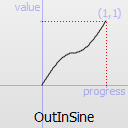Easing.InExpo Easing curve for an exponential (2^t) function: accelerating from zero velocity.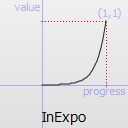Easing.OutExpo Easing curve for an exponential (2^t) function: decelerating from zero velocity.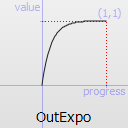Easing.InOutExpo Easing curve for an exponential (2^t) function: acceleration until halfway, then deceleration.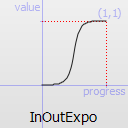Easing.OutInExpo Easing curve for an exponential (2^t) function: deceleration until halfway, then acceleration.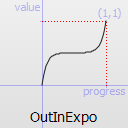Easing.InCirc Easing curve for a circular (sqrt(1-t^2)) function: accelerating from zero velocity.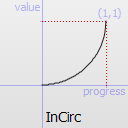Easing.OutCirc Easing curve for a circular (sqrt(1-t^2)) function: decelerating from zero velocity.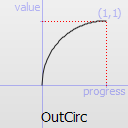Easing.InOutCirc Easing curve for a circular (sqrt(1-t^2)) function: acceleration until halfway, then deceleration.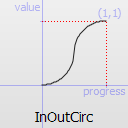Easing.OutInCirc Easing curve for a circular (sqrt(1-t^2)) function: deceleration until halfway, then acceleration.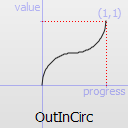Easing.InElastic Easing curve for an elastic (exponentially decaying sine wave) function: accelerating from zero velocity. The peak amplitude can be set with the amplitude parameter, and the period of decay by the period parameter.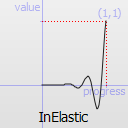Easing.OutElastic Easing curve for an elastic (exponentially decaying sine wave) function: decelerating from zero velocity. The peak amplitude can be set with the amplitude parameter, and the period of decay by the period parameter.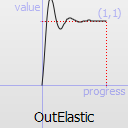Easing.InOutElastic Easing curve for an elastic (exponentially decaying sine wave) function: acceleration until halfway, then deceleration.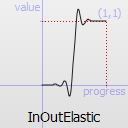Easing.OutInElastic Easing curve for an elastic (exponentially decaying sine wave) function: deceleration until halfway, then acceleration.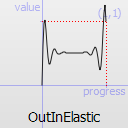Easing.InBack Easing curve for a back (overshooting cubic function: (s+1)*t^3 - s*t^2) easing in: accelerating from zero velocity.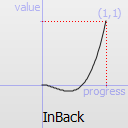Easing.OutBack Easing curve for a back (overshooting cubic function: (s+1)*t^3 - s*t^2) easing out: decelerating to zero velocity.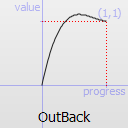Easing.InOutBack Easing curve for a back (overshooting cubic function: (s+1)*t^3 - s*t^2) easing in/out: acceleration until halfway, then deceleration.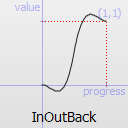Easing.OutInBack Easing curve for a back (overshooting cubic easing: (s+1)*t^3 - s*t^2) easing out/in: deceleration until halfway, then acceleration.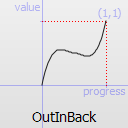Easing.InBounce Easing curve for a bounce (exponentially decaying parabolic bounce) function: accelerating from zero velocity.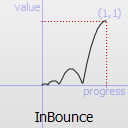Easing.OutBounce Easing curve for a bounce (exponentially decaying parabolic bounce) function: decelerating from zero velocity.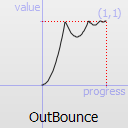Easing.InOutBounce Easing curve for a bounce (exponentially decaying parabolic bounce) function easing in/out: acceleration until halfway, then deceleration.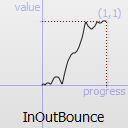Easing.OutInBounce Easing curve for a bounce (exponentially decaying parabolic bounce) function easing out/in: deceleration until halfway, then acceleration.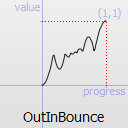easing.amplitude is only applicable for bounce and elastic curves (curves of type Easing.InBounce, Easing.OutBounce, Easing.InOutBounce, Easing.OutInBounce, Easing.InElastic, Easing.OutElastic, Easing.InOutElastic or Easing.OutInElastic).

easing.overshoot is only applicable if easing.type is: Easing.InBack, Easing.OutBack, Easing.InOutBack or Easing.OutInBack.

easing.period is only applicable if easing.type is: Easing.InElastic, Easing.OutElastic, Easing.InOutElastic or Easing.OutInElastic.

See the easing example for a demonstration of the different easing settings.

This property holds the items not to be affected by this animation.

 from : real

This property holds the starting value for the animation.

If the PropertyAnimation is defined within a Transition or Behavior, this value defaults to the value defined in the starting state of the Transition, or the current value of the property at the moment the Behavior is triggered.

 properties : string targets : list

These properties are used as a set to determine which properties should be animated. The singular and plural forms are functionally identical, e.g.

` NumberAnimation { target: theItem; property: "x"; to: 500 }`

has the same meaning as

` NumberAnimation { targets: theItem; properties: "x"; to: 500 }`

The singular forms are slightly optimized, so if you do have only a single target/property to animate you should try to use them.

The targets property allows multiple targets to be set. For example, this animates the x property of both itemA and itemB:

` NumberAnimation { targets: [itemA, itemB]; properties: "x"; to: 500 }`

In many cases these properties do not need to be explicitly specified, as they can be inferred from the animation framework:

 Value Source / Behavior When an animation is used as a value source or in a Behavior, the default target and property name to be animated can both be inferred.``` Rectangle { id: theRect width: 100; height: 100 color: Qt.rgba(0,0,1) NumberAnimation on x { to: 500; loops: Animation.Infinite } //animate theRect's x property Behavior on y { NumberAnimation {} } //animate theRect's y property }``` Transition When used in a transition, a property animation is assumed to match all targets but no properties. In practice, that means you need to specify at least the properties in order for the animation to do anything.``` Rectangle { id: theRect width: 100; height: 100 color: Qt.rgba(0,0,1) Item { id: uselessItem } states: State { name: "state1" PropertyChanges { target: theRect; x: 200; y: 200; z: 4 } PropertyChanges { target: uselessItem; x: 10; y: 10; z: 2 } } transitions: Transition { //animate both theRect's and uselessItem's x and y to their final values NumberAnimation { properties: "x,y" } //animate theRect's z to its final value NumberAnimation { target: theRect; property: "z" } } }``` Standalone When an animation is used standalone, both the target and property need to be explicitly specified.``` Rectangle { id: theRect width: 100; height: 100 color: Qt.rgba(0,0,1) //need to explicitly specify target and property NumberAnimation { id: theAnim; target: theRect; property: "x"; to: 500 } MouseArea { anchors.fill: parent onClicked: theAnim.start() } }```

As seen in the above example, properties is specified as a comma-separated string of property names to animate.

 to : real

This property holds the end value for the animation.

If the PropertyAnimation is defined within a Transition or Behavior, this value defaults to the value defined in the end state of the Transition, or the value of the property change that triggered the Behavior.

Publicité

## Best Of

Semaine
Mois
Année

### Le Qt Quarterly au hasard#### Le repérage des paires de parenthèses avec QSyntaxHighlighter

Qt Quarterly est la revue trimestrielle proposée par Nokia et à destination des développeurs Qt. Ces articles d'une grande qualité technique sont rédigés par des experts Qt. Lire l'article.

### Contact

• Vous souhaitez rejoindre la rédaction ou proposer un tutoriel, une traduction, une question... ? Postez dans le forum Contribuez ou contactez-nous par MP ou par email (voir en bas de page).

### Qt dans le magazine

 Cette page est une traduction d'une page de la documentation de Qt, écrite par Nokia Corporation and/or its subsidiary(-ies). Les éventuels problèmes résultant d'une mauvaise traduction ne sont pas imputables à Nokia. Qt 4.7 Copyright © 2012 Developpez LLC. Tous droits réservés Developpez LLC. Aucune reproduction, même partielle, ne peut être faite de ce site et de l'ensemble de son contenu : textes, documents et images sans l'autorisation expresse de Developpez LLC. Sinon, vous encourez selon la loi jusqu'à 3 ans de prison et jusqu'à 300 000 E de dommages et intérêts. Cette page est déposée à la SACD. Vous avez déniché une erreur ? Un bug ? Une redirection cassée ? Ou tout autre problème, quel qu'il soit ? Ou bien vous désirez participer à ce projet de traduction ? N'hésitez pas à nous contacter ou par MP !

Partenaires

Hébergement Web